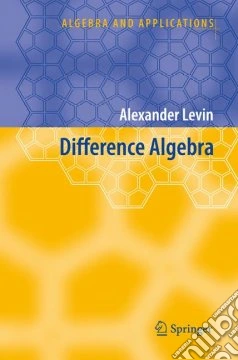ricerca
avanzata

# Difference Algebra

## Un libro in lingua di Alexander Levin edito da Springer Verlag, 2008

• € 114,90
• Il prezzo è variabile in funzione del cambio della valuta d’origineDifference algebra grew out of the study of algebraic difference equations with coefficients from functional fields. The first stage of this development of the theory is associated with its founder, J.F. Ritt (1893-1951), and R. Cohn, whose book Difference Algebra (1965) remained the only fundamental monograph on the subject for many years. Nowadays, difference algebra has overgrown the frame of the theory of ordinary algebraic difference equations and appears as a rich theory with applications to the study of equations in finite differences, functional equations, differential equations with delay, algebraic structures with operators, group and semigroup rings.The monograph is intended for graduate students and researchers in difference and differential algebra, commutative algebra, ring theory, and algebraic geometry. The book is self-contained; it requires no prerequisites other than the knowledge of basic algebraic concepts and a mathematical maturity of an advanced undergraduate.